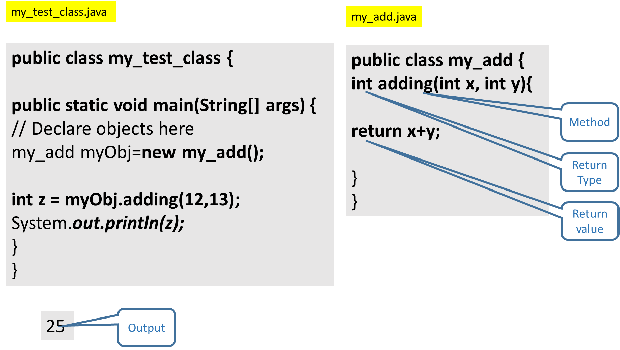# Java Object Oriented Programming

Check this code to indentify attribute and object. How we can access one attribute ?
``````package my_proj;

public class my_first {
int i=20; // attribute created.

public static void main(String[] args) {
my_first my_num=new my_first(); // Object Created
System.out.println(my_num.i); // 20
}

}``````
In above code we have created one variable int i =20;.
This variable is also known as attribute of the class my_first.

## object

Check this line of above code.
``my_first my_num=new my_first();``
in above code we creates an object by name my_num. We can say my_num is an object of class my_first.
Objects can inherit all the variables and methods of the class.

## object and attribute

We can access any attribute of the class my_first by using dot ( . ) notation. In the last line we have used our object my_num and dot notation to get the value of attribute i of my_first. Output is 20 here.
``System.out.println(my_num.i); // 20 ``

## method

We have created attributes ( variables within a class ) and accessed the same by using objects of the class in above code. Now we will create methods inside a class. By using methods we can perform some tasks. ( try to understand difference between attribute and method )

Let us try to add two numbers by using one method and name it as adder. We will create an object of the class my_first and use the same to execute the method. Here is the code.
``````package Pack1;
class my_first {
int i =20;
int j=10;
public void adder() { // Method is created.
System.out.println(i+j);
}

public static void main(String args[])
{
my_first obj1= new my_first();
System.out.println(obj1.i);//20
obj1.j=40;
}

}``````
In above example you can identify the following points.

attributes, class, method, Object

Check this line
``obj1.j=40; ``
Here we have overriding the existing value of the attribute. Now the value of j=40 ( not 10 ). The output of above code after using the method adder() is 60.

## final

In above code we have updated the value of attribute j to 40 by using our object obj1. We may not allow this updating or everriding of attributes by using final while declaring the attribute. Update this line like this.
``final int j=10;``
Now we can't update the value of j to any other value. This the exceptions we will get when we try to update the value of j.
`` The final field my_first.j cannot be assigned``
We can create class in a separate file in same package and use the class.
``````package Pack1;

class my_first {
int i =20;
int j;
{
System.out.println(i+j); // 50
}
public static void main(String args[])
{
my_first obj1 = new my_first();
System.out.println(obj1.i); // 20
obj1.j=30;
}

}``````
my_second.java
``````package Pack1;

public class my_second {
public static void main(String args[])
{
my_first obj2 = new my_first();
obj2.j=10;
}

}``````
This way we can use different methods.

Let us try one class where we can use addition , subtraction , multiplication and division of two numbers.

my_second.java
``````package Pack1;

public class my_second {
public static void main(String args[])
{
my_first obj2 = new my_first();
obj2.j=20;
obj2.i=40;
obj2.my_subtract();
obj2.my_multiply();
obj2.my_devide();

}

}``````
my_first.java
``````package Pack1;
class my_first {
int i =20;
int j;
{
System.out.println(i+j);
}

public void my_subtract() {
System.out.println(i-j);
}
public void my_multiply() {
System.out.println(i*j);
}
public void my_devide() {
System.out.println(i/j);
}
public static void main(String args[])
{

}

}``````
The output is here
``````60
20
800
2``````

## public and private methods

In above code if we change any method to private then we will get exceptions like this

The method my_subtract() from the type my_first is not visible
``````private void my_subtract() {
System.out.println(i-j);
}``````

## static and public methods

We can access any public method by using object, however we can access any static method without creating any object.

Here my_static is an static object so we can access it directly. However my_public() is an public method so we need to create an object ( obj1 ) to access my_public() method.
``````package Pack1;

public class my_second {
static void my_static(){
//System.out.println(i*j);
System.out.println("Hi this is my_static");
}
public void my_public() {
System.out.println("Hi this is my_public");

}
public static void main(String args[])
{
//my_public(); // this will generate Exception
my_second obj1=new my_second(); // Object is created
obj1.my_public();    // method is executed.

// Can access without object
my_static();
}
}
``````
Output
``````Hi this is my_public
Hi this is my_static``````

## Examplemy_test_class.java
``````public class my_test_class {

public static void main(String[] args) {
// Declare objects here

System.out.println(z);

}

}``````
``````public class my_add {

return x+y;
}
}``````

## Subscribe

* indicates required
Subscribe to plus2netplus2net.com